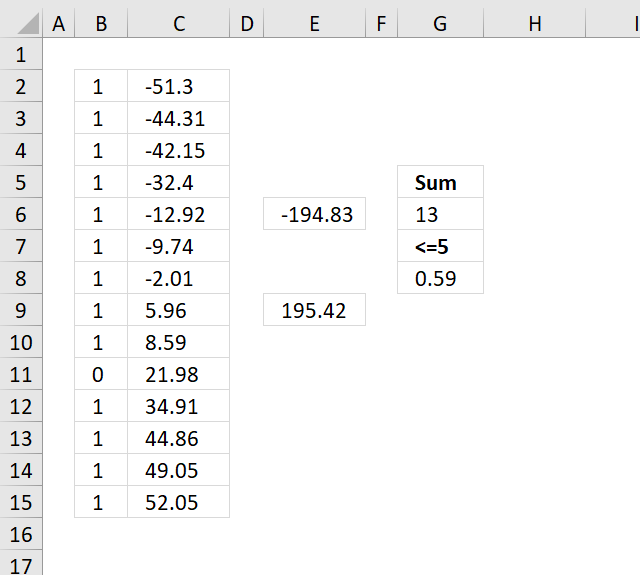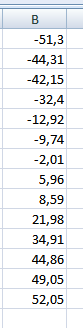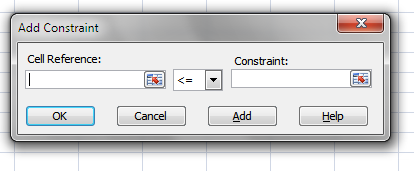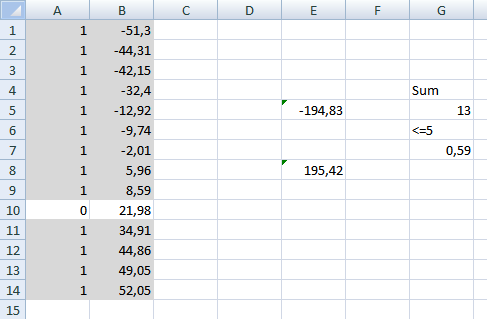Author: Oscar Cronquist Article last updated on December 11, 2018I found this excel question:

I am a Controller in a multinational company. We have many transactions (sales, credits, debits, etc.) with related parties but seldom send payments. Once in a while, I would like to reduce the number of open items by identifying positive and negative amounts that net to zero and clear those items.

Currently, I dump the open items into Excel, sort by value, and manually play with items until I get a combination that is nearly zero (it seems we never find a combination that is exactly zero).

Since my goal is to minimize the number of open items, not only do I need to net as close to zero as possible (a few dollars negative or positive can be simply written off), but I also try to include as many transactions as possible in the final answer. That is, netting two transactions for +5 and -5 is fine but netting four transactions for +1, +2, +2 and -5 is better.

The following article solves this question using an array formula:

Find numbers closest to sum

Excelxor is such a great website for inspiration, I am really impressed by this post Which numbers add up to […]

The answer here uses the Excel Solver, here are some random numbers that will work as positive and negative amounts.The maximum difference between possible sums is 5\$ (G7). I want Excel solver to find the maximum number of positive and negative amounts that sums to almost zero. (G5).

Formula in E5:

=SUMPRODUCT(A1:A7;B1:B7) + ENTER

Formula in E8:

=SUMPRODUCT(A8:A14;B8:B14) + ENTER

Formula in G7:

=SUM(A1:A7)+SUM(A8:A14) + ENTER

Formula in G5:

=ABS(E5+E8) + ENTER

If you already have Excel 2007 Solver add-in installed, skip next section.

1. Click Office button
2. Click Excel Options
4. Install Solver
5. Click OK

### Start Excel 2007 Solver

1. Click "Data" in the Ribbon
2. Click "Solver"3. Click "Set Target Cell:" button
4. Click G7
5. Click Max in "Equal to:".
6. Click "By Changing Cells:" button and select A1:A14.8. Click "Cell Reference:" button and select A1:A14
9. Select "bin" in drop down menu (triangle)